## The Final exam

The final exam took place August 4, 2016 at the usual time in the usual classroom. We had a global review on August 2, 2016. The average was 79/100 the standard deviation was 16 (when the exam is scaled to 100), both exactly like for the second midterm. The maximal achieved score was 137.
 The exam Solution draft

Some practice exams: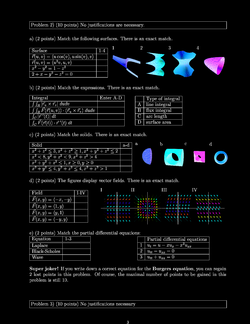Practice I, [Solutions]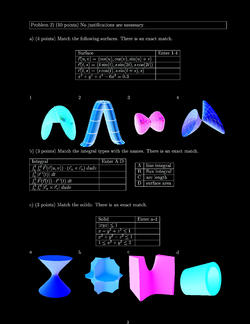Practice II, [Solutions]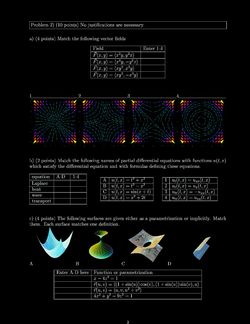Practice III, [Solutions]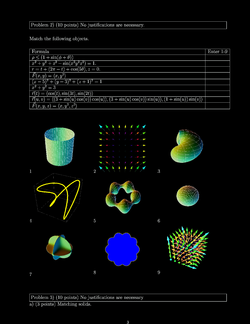Practice IV, [Solutions]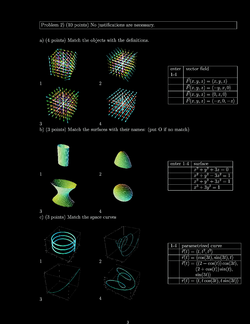Practice V, [Solutions]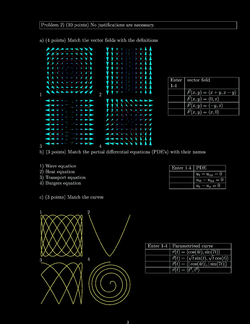Practice VI, [Solutions]

## Second hourly

The second hourly took place on July 21, 2016. We had a class review on July 19, 2016. The average score was 79. The standard deviation was 16. Again the maximal score was 99.
 The exam Solution draft

Here are some practice exams: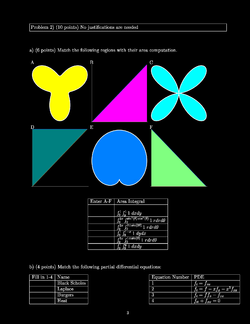Practice I, [Solutions]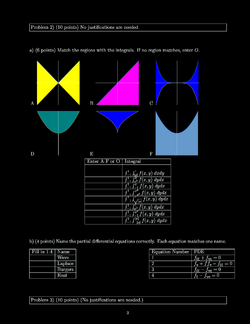Practice II, [Solutions]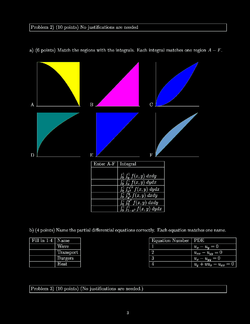Practice III, [Solutions]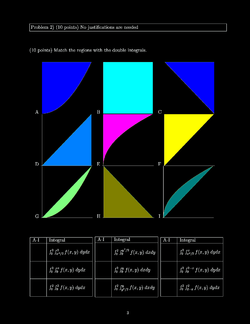Practice IV, [Solutions]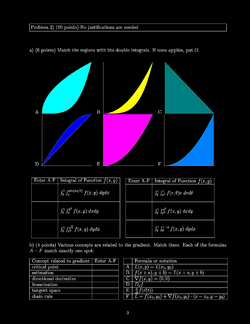Practice V, [Solutions]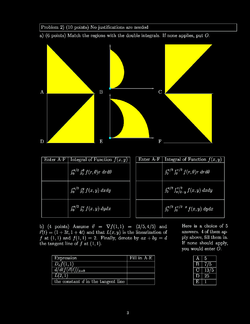Practice VI, [Solutions]

## First hourly

The first hourly took place on July 7, 2016. We had a review in class on July 5, 2016. The papers will be returned on Tuesday. The average score was 78. The standard deviation 18. The maximal score achieved was 99.
 The exam Solution draft

Here are some practice exams: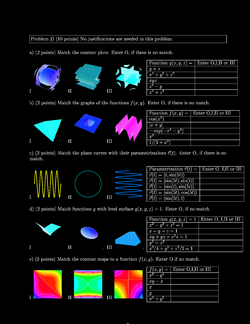Practice I, [Solutions]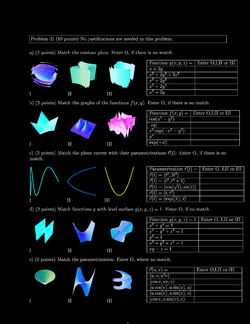Practice II, [Solutions]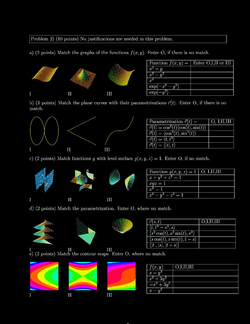Practice III, [Solutions]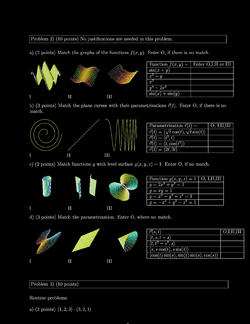Practice IV, [Solutions]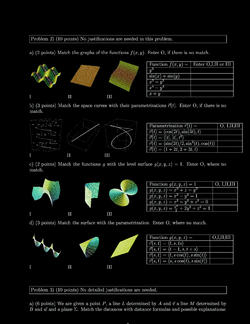Practice V, [Solutions]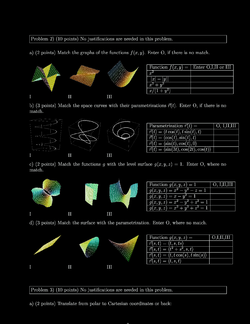Practice VI, [Solutions]